# Feistel cipher facts for kids

Kids Encyclopedia Facts

In cryptography, a Feistel cipher is a symmetric structure used in the construction of block ciphers, named after the German IBM cryptographer Horst Feistel; it is also commonly known as a Feistel network. A large set of block ciphers use the scheme, including the Data Encryption Standard

The Feistel structure has the advantage that encryption and decryption operations are very similar, even identical in some cases, requiring only a reversal of the key schedule. Therefore the size of the code or circuitry required to implement such a cipher is nearly halved.

Feistel construction is iterative in nature which makes implementing the cryptosystem in hardware easier.

Feistel networks and similar constructions are product ciphers, and so combine multiple rounds of repeated operations, such as:

Bit shuffling creates the diffusion effect, while substitution is used for confusion.

## Theoretical work

Many modern symmetric block ciphers use Feistel networks, and the structure and properties of Feistel ciphers have been extensively explored by cryptographers. Specifically, Michael Luby and Charles Rackoff analyzed the Feistel block cipher construction, and proved that if the round function is a cryptographically secure pseudorandom function, with Ki used as the seed, then 3 rounds is sufficient to make the block cipher a pseudorandom permutation, while 4 rounds is sufficient to make it a "strong" pseudorandom permutation (which means that it remains pseudorandom even to an adversary who gets oracle access to its inverse permutation). Because of this very important result of Luby and Rackoff, Feistel ciphers are sometimes called Luby-Rackoff block ciphers. Further theoretical studies generalized the construction, and defined more precise limits for security.

## Construction

Let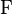${\rm F}$ be the round function and let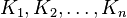$K_1,K_2,\ldots,K_{n}$ be the sub-keys for the rounds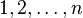$1,2,\ldots,n$ respectively.

Then the basic operation is as follows:

Split the plaintext block into two equal pieces, (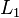$L_1$,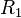$R_1$)

For each round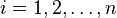$i =1,2,\dots,n$, compute (calculate)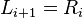$L_{i+1} = R_i\,$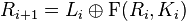$R_{i+1}= L_i \oplus {\rm F}(R_i, K_i)$.

Then the ciphertext is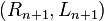$(R_{n+1}, L_{n+1})$. (Commonly the two pieces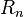$R_n$ and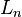$L_n$ are not switched after the last round.)

Decryption of a ciphertext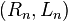$(R_n, L_n)$ is accomplished by computing for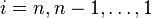$i=n,n-1,\ldots,1$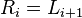$R_{i} = L_{i+1}\,$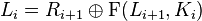$L_{i} = R_{i+1} \oplus {\rm F}(L_{i+1}, K_{i})$.

Then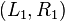$(L_1,R_1)$ is the plaintext again.

One advantage of this model is that the round function${\rm F}$ does not have to be invertible, and can be very complex.

The diagram illustrates the encryption process. Decryption requires only reversing the order of the subkey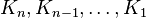$K_{n},K_{n-1},\ldots,K_1$ using the same process; this is the only difference between encryption and decryption:

Unbalanced Feistel ciphers use a modified structure where$L_1$ and$R_1$ are not of equal lengths. The MacGuffin cipher is an experimental example of such a cipher.

The Feistel construction is also used in cryptographic algorithms other than block ciphers. For example, the Optimal Asymmetric Encryption Padding (OAEP) scheme uses a simple Feistel network to randomize ciphertexts in certain asymmetric-key encryption schemes.

## List of Feistel ciphers

Feistel or modified Feistel: Blowfish, Camellia, CAST-128, DES, FEAL, ICE, KASUMI, LOKI97, Lucifer, MARS, MAGENTA, MISTY1, RC5, TEA, Triple DES, Twofish, XTEA, GOST 28147-89

Generalised Feistel: CAST-256, MacGuffin, RC2, RC6, SkipjackFeistel cipher Facts for Kids. Kiddle Encyclopedia.